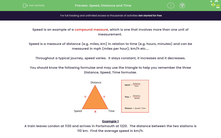# Speed, Distance and Time

In this worksheet, students work out average speeds, total distances and total times taken in the given journeys.Key stage:  KS 3

Curriculum topic:   Ratio, Proportion and Rates of Change

Curriculum subtopic:   Solve Problems Involving Compound Units

Difficulty level:#### Worksheet Overview

Speed is an example of a compound measure, which is one that involves more than one unit of measurement.

Speed is a measure of distance (e.g. miles, km) in relation to time (e.g. hours, minutes) and can be measured in mph (miles per hour), km/h etc....

Throughout a typical journey, speed varies.  It stays constant, it increases and it decreases.

You should know the following formulae and may use the triangle to help you remember the three Distance, Speed, Time formulae.Example 1

A train leaves London at 1130 and arrives in Portsmouth at 1220.  The distance between the two stations is 110 km.  Find the average speed in km/h.

First we must state the time taken in hours, not in minutes.

Time taken = 50 minutes = 5/6 hour

Average Speed = Total distance ÷ Total time = 110 ÷ 5/6 = 110 × 6/5 = 132 km/h

Example 2

During a car journey, John travels at an average speed of 90 km/h for 2 hours 30 minutes.  Find the distance travelled.

Distance = Speed x Time

The units must match so time must be measured in hours.

Distance = 90 x 2½ = 225 km

### What is EdPlace?

We're your National Curriculum aligned online education content provider helping each child succeed in English, maths and science from year 1 to GCSE. With an EdPlace account you’ll be able to track and measure progress, helping each child achieve their best. We build confidence and attainment by personalising each child’s learning at a level that suits them.

Get started